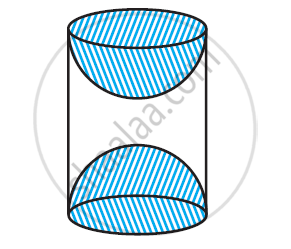Share

# A wooden article was made by scooping out a hemisphere from each end of a solid cylinder, as shown in given figure. If the height of the cylinder is 10 cm - CBSE Class 10 - Mathematics

ConceptSurface Area of a Combination of Solids

#### Question

A wooden article was made by scooping out a hemisphere from each end of a solid cylinder, as shown in given figure. If the height of the cylinder is 10 cm, and its base is of radius 3.5 cm, find the total surface area of the article [Use π = 22/7]#### Solution

Given that,

Radius (r) of cylindrical part = Radius (r) of hemispherical part = 3.5 cm

Height of cylindrical part (h) = 10 cm

Surface area of article = CSA of cylindrical part + 2 × CSA of hemispherical part

=2πrh + 2 x ππr2

2π x 3.5 x 1+ + 2 x 2π x 3.5 x 3.5

= 70π+ 49π

= 119π

= 17 x 22 = 374 cm2

Is there an error in this question or solution?

#### Video TutorialsVIEW ALL 

Solution A wooden article was made by scooping out a hemisphere from each end of a solid cylinder, as shown in given figure. If the height of the cylinder is 10 cm Concept: Surface Area of a Combination of Solids.
S